GeeksforGeeks App
Open AppBrowser
Continue

# CBSE Class 12 Chemistry Previous Year Question Paper 2022

The CBSE Class 12 Previous Year’s Question Paper for Physics is a resourceful tool from GfG to empower students in their exam preparation journey. With the help of this question, paper students can get insights about the examinations such as the pattern of examinations, weightage of chapters and units, and also the understanding of solution writing.
This Previous year’s question paper comes with a solution, so that students after completing the revision of the complete syllabus, can evaluate their performance using the previous year’s paper. By comparing their solutions to the ideal solution provided by GfG, students can better understand the weak areas of their preparation. Thus, the CBSE Class 12 Previous year Question Paper is the most beneficial tool for the preparation for the board examination.

## SECTION A

### Q1: Define rate of reaction. Write two factors that affect the rate of reaction.

Rate of reaction is defined as decrease in concentration of reactant with time or increase in concentration of product with time. It is often said to be the speed of reaction. Rate of reaction is usually calculated in the terms of concentration of product consumed in a reaction or concentration of reactants formed in the reaction.

The two Factors that affect rate of reaction are as follows:

• Concentration of the Reactants
• Temperature

For more on Factors Affecting Rate of a Reaction

### (i) In decreasing order of basic strength in aqueous solution: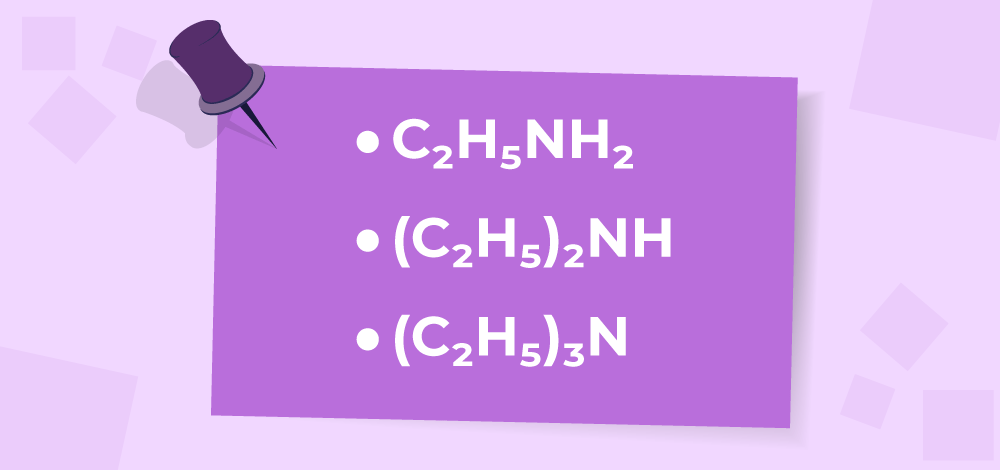### (ii) In increasing order of solubility in water: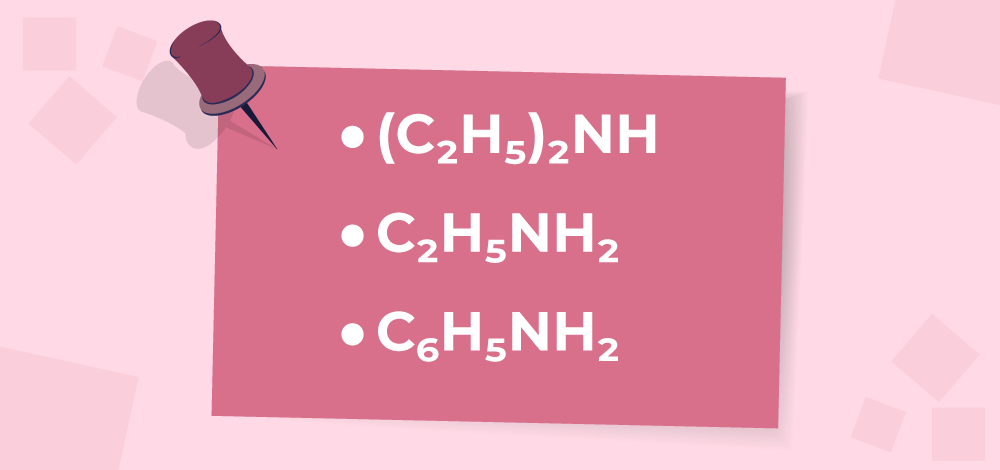### (iii) In decreasing order of their pKb values: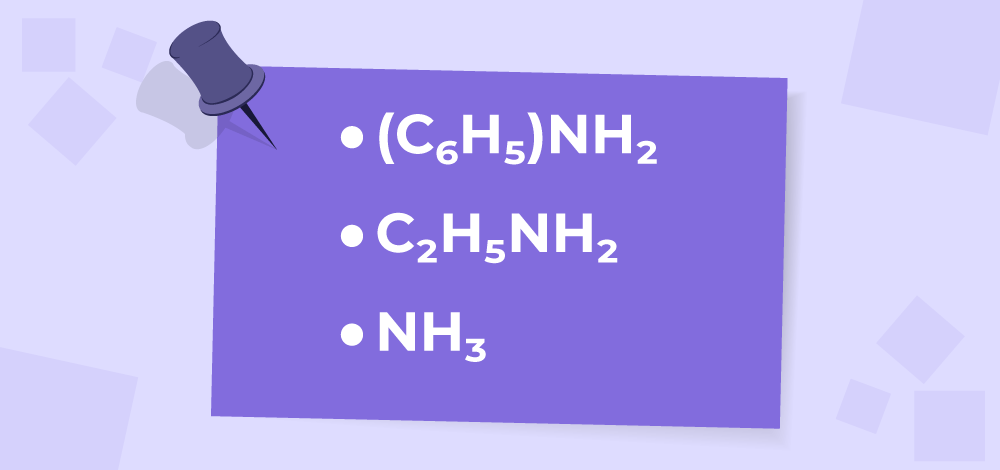(i) The basic strength of a compound in aqueous solution depends on the ability of the compound to donate a lone pair of electrons to a proton. The greater the ability, the stronger the base. Thus, the decreasing order of basic strength would be:

(C2H5)2 NH > (C2H5)N > C2H5NH2

(ii) The solubility of a compound in water depends on its polarity and ability to form hydrogen bonds with water molecules. Thus, the increasing order of solubility in water would be:

C6H5NH2 < (C2H5)2NH < C2H5NH2

(iii) pKb is a measure of the strength of a base. The lower the pKb, the stronger the base. Thus, the decreasing order of pKb values would be:

C6H5NH2 > NH3 > C2H5NH2

### (ii) Zn 2+ ions is increased

Nernest Equation for Zn(s) + Cu2+ (aq) ➔ Zn2+ (aq) + Cu(s)  is,

Ecell = E0cell – 0.059/2 log [Zn2+]/[Cu2+]

Ecell = E°cell – (RT/nF) × ln(Q)

where,
E°cell = standard cell potential
R = gas constant (8.314 J/K×mol)
T = temperature in Kelvin
n = number of electrons transferred in the cell reaction
F = Faraday constant (96,485 C/mol)
Q = reaction quotient

(i) Ecell will increase

When the concentration of Cu2+ ions increase, it will result in an increase of cell potential (Ecell). This is because the concentration of Cu2+ in the denominator equation becomes larger, making the natural logarithm term more positive and subtracting less from the positive E°cell term.

(ii) Ecell will decrease

On contrary, when Zn2+ ion concentrations are increased, the Ecell value decreases as a result of an increased concentrate on Zn2+ in the numerator that results in a larger value of/. This makes the natural logarithm term more negative and subtracting more from the positive E°cell term.

## SECTION B

### Give suitable reasons for each.

(i) Cr3+, due to stable t23g configuration. The most stable ions are those with a full outermost shell of electrons, such as the Cr3+ ion. This is because charged ions have electrostatic potential energy, and the most stable configurations are those that minimize this energy.

(ii) Mn3+, as Mn is more stable in +2 oxidation state / stable half-filled d5 configuration of Mn2+. An ion with a higher positive oxidation state tends to be a stronger oxidizing agent. Among the given ions, Mn3+ has the highest positive oxidation state, and it can easily be oxidized to Mn2+ or MnO2. Therefore, Mn3+ would be the strongest oxidizing agent among the given ions.

(iii) Ti4+, no unpaired electrons / no d-d transition / d0 configuration. Transition metal ions in aqueous solution exhibit characteristic colours due to the d-d electronic transitions. An ion that does not have any unpaired electrons in the d-orbitals or that has all the d-electrons paired would be colourless. Among the given ions, Ti4+ has all the d-electrons paired in the d-orbitals, and hence it would be colourless in aqueous solution

### (iii) IUPAC name of the complex.

(a)

(i) t23geg1.

Crystal Field Splitting Energy (CFSE), or Δo is the energy separation between two splitted d-orbitals in a crystal field. This phenomenon arises when ligands interact with a central metal ion and cause the degenerate d-orbitals to separate, which is called crystal field splitting. The geometry of these ligands determines the amount of CFSE.

(ii) d sp2, diamagnetic

According to valence bond theory, the hybridization of [Ni(CN)4]2- is dsp2. Each CN ligand donates a lone pair of electrons to the Ni atom, forming a sigma bond with one of the hybridized orbitals.

To know the magnetic character of the complex, we find the number of unpaired electrons. The nickel ion contains 28 electrons, with the 3d8 4s2 configuration. In the [Ni(CN)4]2-complex, the nickel ion loses two electrons to form the +2 oxidation state, giving it a 3d8 configuration. As a result, all the electrons are paired in the t2g set, and there are no unpaired electrons. Therefore, the complex is diamagnetic.

(iii)

Dichloridobis (ethane-1,2-diamirte) cobalt is [CoCl2(en)2]+. The formula for the complex would be: CoCl2(NH2CH2CHNH2)2, as per IUPAC norms.

### OR

(b)

(i) The structural formula is given as  [Ni(H2O6)]Cl2.

(ii) 6

The secondary valency of ‘Ni’ in the complex is 6, as indicated by the formula NiCl2.

(iii)

Hexaaquanickel (II) Chloride

The complex contains a nickel(II) ion coordinated to six water molecules in an octahedral arrangement. The chloride ions are present as counter ions to balance the charge of the complex. When the complex is mixed with silver nitrate, the silver ions react with the chloride ions to form insoluble AgCl precipitate.

### Q6. A first-order reaction is 50% complete in 40 minutes. Calculate the time required for the completion of 90% of the reaction.

[Given: log 2 = 0.3010, log 10 = 1]

The equation t1/2 = 0.693/k is the mathematical relationship between the half-life (t1/2) of a first-order reaction and the rate constant (k) of the reaction.

t1/2 = 0.693/k

k = 0.693/t1/2 = 0.693/40 min-1

The equation t = 2.303/k log [R0]/[R] is a mathematical relationship that relates the time (t) required for a reaction to reach completion to the initial concentration ([R0]) of the reactant and the concentration ([R]) of the reactant at any given time during the reaction.

For 90% completion

By,

t = 2.303/k log [R0]/[R]
= 2.303/0.693 × 40 × log 100/10
= 2.303/0.693 × 40
= 132.9 min

### (b) Write the structures of A, Band C in the following reactions: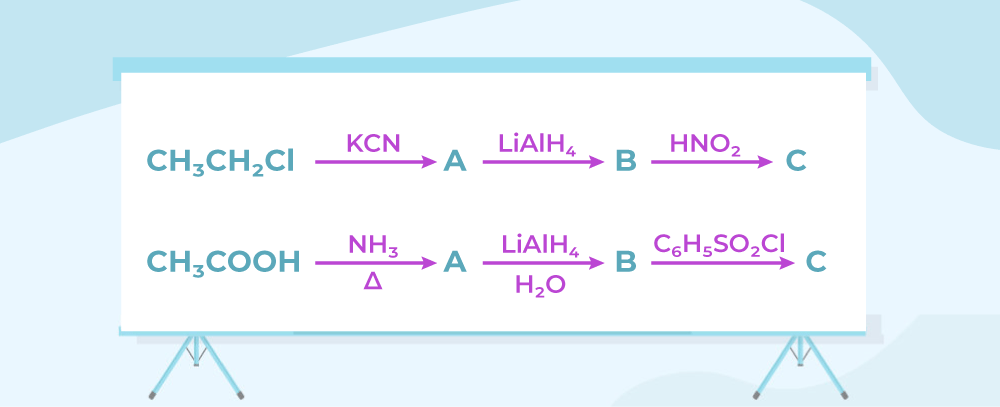(i)

The Gabriel Phthalimide synthesis is a method for the synthesis of primary amines from phthalimide and alkyl halides. N-alkylphthalimide is formed followed by hydrolysis to release the main amine.

The following picture depicts the Gabriel Phthalimide Synthesis: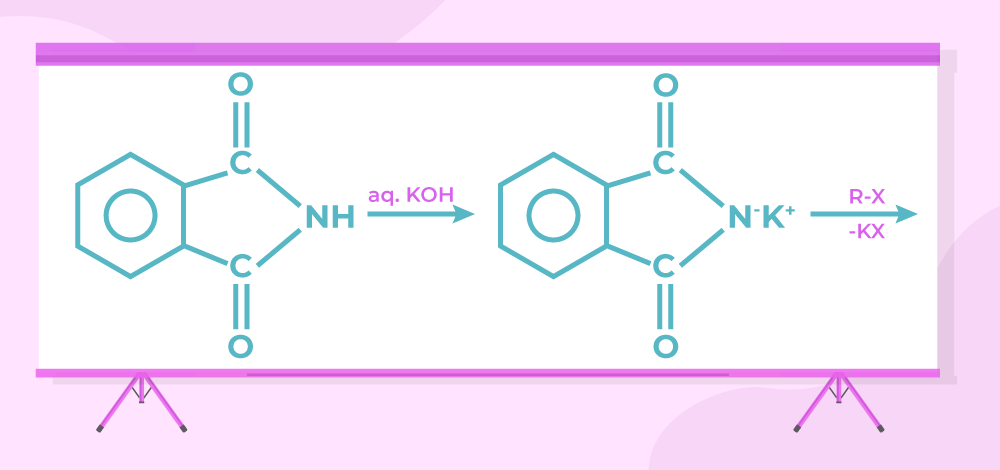(ii)

In this reaction, the primary amine reacts with trichloromethane(chloroform) in the presence of alcoholic potassium hydroxide (KOH) to produce carbylamine.

The following picture depicts the Carbylamine Reaction: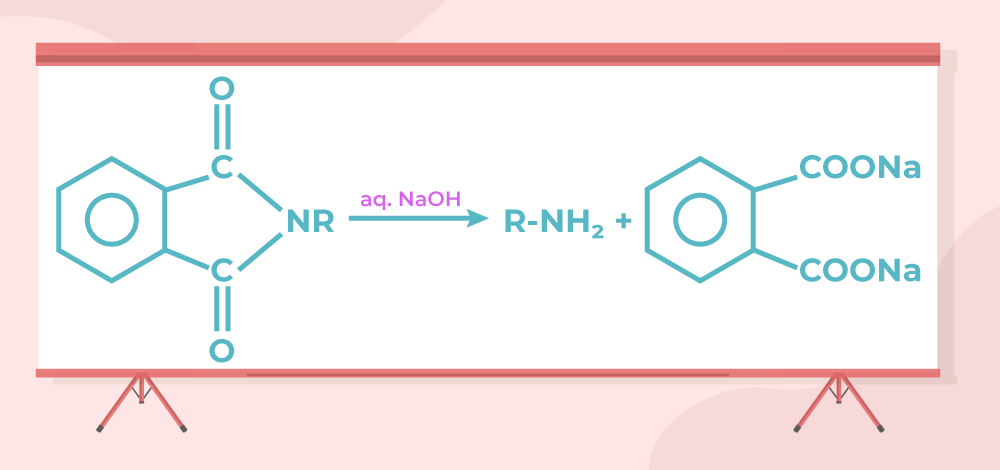(iii)

Hofmann-Bromamide degradation reaction is a chemical reaction to convert primary amides into primary amines. During the reaction, the amide group is converted to an isocyanate intermediate, which is afterwards hydrolyzed to produce the main amine. It includes reaction of amide with bromine and sodium hydroxide and then treated with aqueous solution.

The following picture depicts the Hofmann-Bromamide degradation reaction: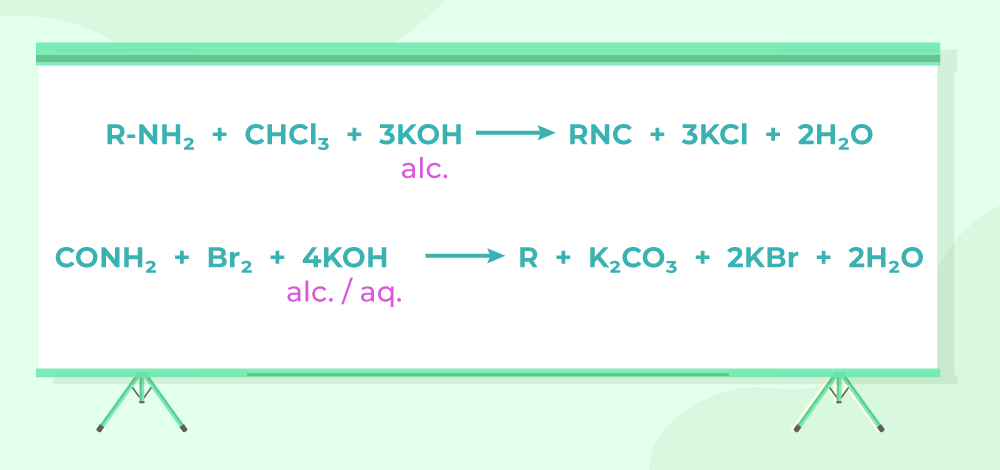### OR

(i)

In the first reaction, A is Propionitrile  which in the presence of potassium cyanide gives Propylamine Propyl, which further gives C Propanol.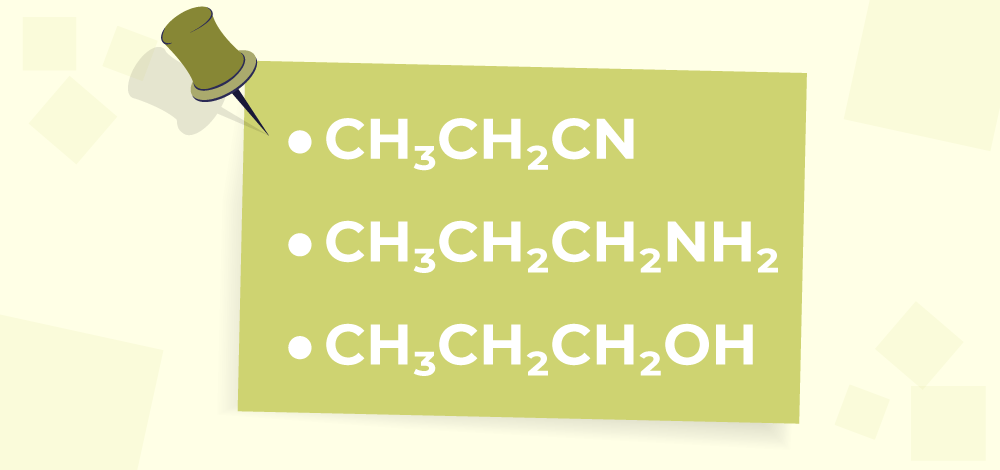(ii)

In this reaction, Acetic acid reacts with ammonia to give A Acetamide, which gives B on reacting with Lithium tetrahydridoaluminate. So B is Ethylamine. Then Ethylamine reacts with a tertiary amine to produce C.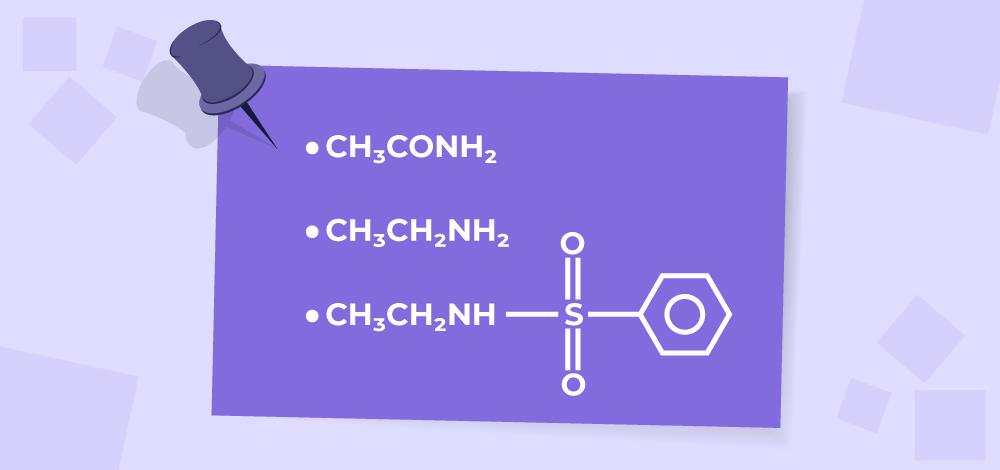### (b) Define transition elements. Which of the d-block elements may not be regarded as the transition· elements? Why do transition metals generally form coloured compounds?

(a) (i)

Because of greater number of unpaired electrons which cause strong metallic bonding / strong interatomic interaction forces. The transition elements have higher enthalpies of atomization because they have partially filled d-orbitals. These d-orbitals are located in the middle of the electron shell and are shielded by the outer s and p orbitals. The electrons in the d-orbitals are therefore held more tightly by the nucleus, resulting in higher enthalpies of atomization.

(a) (ii)

Because of lanthanoid contraction / similar atomic or ionic radii / similar properties. The separation of a mixture of Lanthanoid elements is difficult due to the similar sizes and chemical properties of the lanthanoids. This makes it challenging to separate them using traditional chemical separation methods. In addition, the lanthanoids have very similar electronic configurations, which makes it difficult to distinguish between them using chemical reactions.

(a) (iii)

Because of high ΔaH0  and low ΔhydH0 The positive value of E0 M2+/M for copper indicates that it is more likely to be reduced than

hydrogen.

### OR

(b)

Transition elements, also known as transition metals, are elements in the d-block of the periodic table. They have partially filled d-orbitals in their valence electronic configuration and exhibit characteristic properties such as variable oxidation states, complex formation, and catalytic activity.

The d-block elements that are not considered transition elements are Zn, Cd, and Hg. This is because they have completely filled d-orbitals in their valence electronic configuration and do not exhibit the characteristic properties of transition elements.

Transition metals generally form colored compounds due to the presence of partially filled d-orbitals in their electronic configuration. When a transition metal ion is present in a compound, some of its electrons are promoted from the lower energy d-orbitals to higher energy d-orbitals when it absorbs light. The energy absorbed by the electrons corresponds to the energy of light in the visible region of the electromagnetic spectrum, causing the compound to appear colored.

### Q9. An organic compound ‘X’ with the molecular formula C5H10O forms a 2,4-DNP derivative, does not reduce Tollens’ reagent but gives a positive iodoform test on heating with I2 in the presence of NaOH. Compound ‘X’ gives ethanoic acid and propanoic acid on vigorous oxidation. Write the (i) Structure of the compound ‘X’. (ii) Structure of the product obtained when compound ‘X’ reacts with 2,4-DNP ‘reagent. (iii) Structures of the products obtained when compound ‘X’ is heated with I2 in the presence of NaOH.

(i)

X: Methyl Propyl Ketone or Pentanone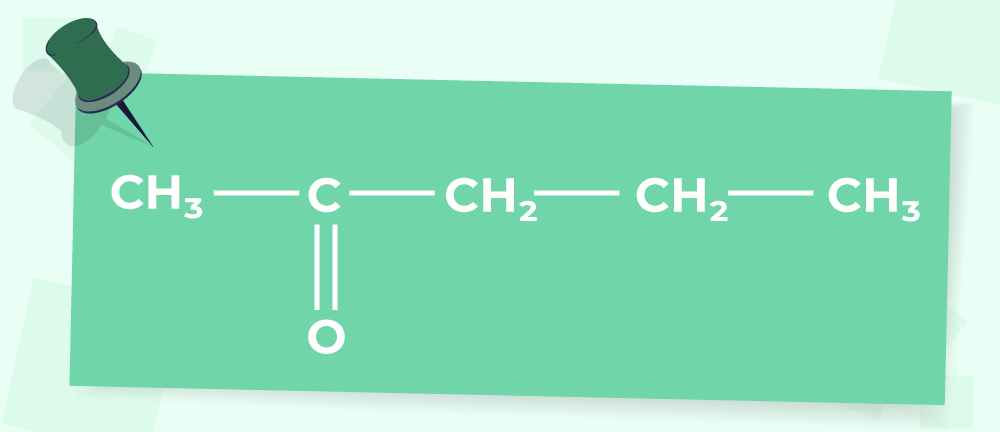(ii)

‘X’ reacts with 2,4-DNP ‘reagent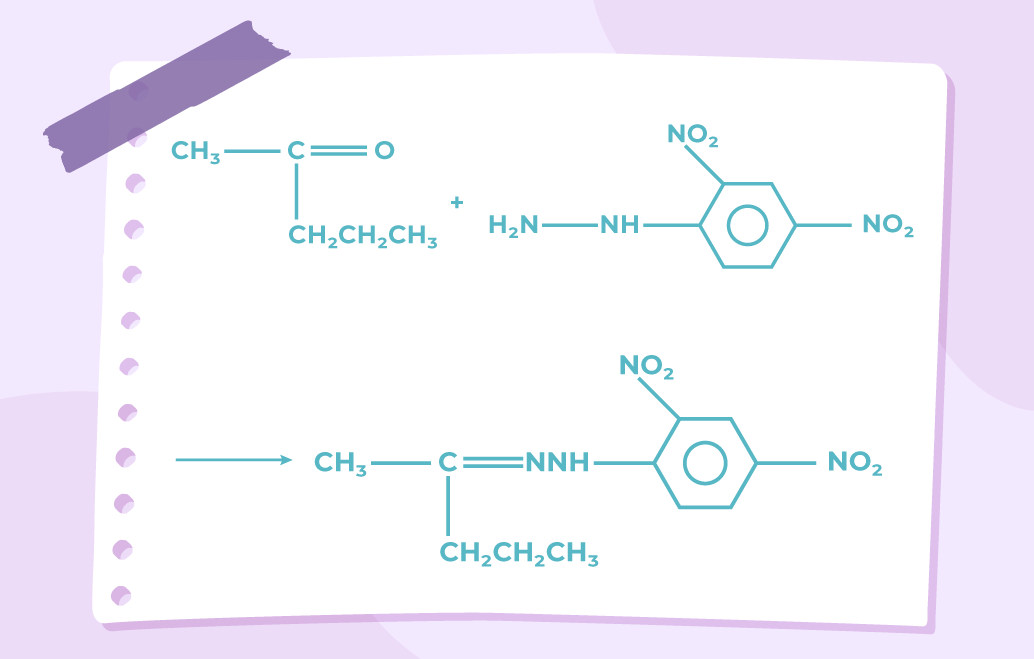(iii)

‘X’ is heated with I2 in the presence of NaOH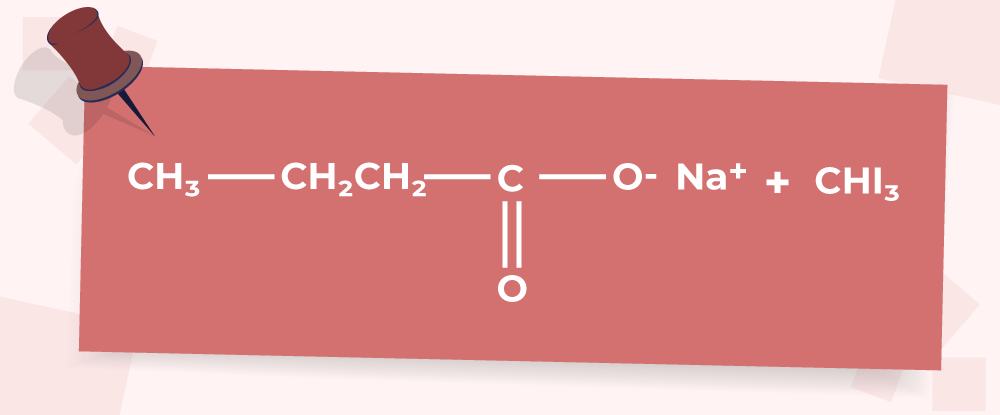### (iii) Coagulation

(a) Differences are as follows,

• Physisorption is a physical adsorption process that involves weak van der Waals forces, such as London dispersion forces, dipole-dipole interactions, and hydrogen bonding, between the adsorbate and adsorbent.
• In contrast, chemisorption is a chemical adsorption process that involves strong chemical bonds, such as covalent or ionic bonds, between the adsorbate and adsorbent.

• The energy of adsorption in physisorption is between 20-40 kJ/mol which is comparatively low.
• The energy of adsorption in chemisorption is between 80-400 kJ/mol which is high.

• Physisorption is a reversible process.
• Chemisorption is an irreversible process.

### OR

(b) (i)

Lyophilic sol is a type of colloid in which the dispersed phase (solid or liquid) has a strong affinity for the dispersion medium (usually a liquid). This strong affinity results in the formation of a stable sol that does not readily settle out or coagulate. An example of a lyophilic sol is a starch sol, in which starch molecules form a stable dispersion in water due to their ability to form hydrogen bonds with water molecules.

(b) (ii)

Macromolecular colloid is a type of colloid in which the dispersed phase consists of large molecules or macromolecules. These macromolecules can be either natural or synthetic polymers. A common example of a macromolecular colloid is a protein sol.

(b) (iii)

Coagulation is the process by which a colloid is destabilized and the dispersed phase aggregates to form larger particles that settle out of the dispersion medium. This process can be induced by various factors, such as the addition of an electrolyte or a change in pH or temperature. An example of coagulation is the addition of salt to a gold sol.

### (b) Calculate λm0 for MgCl2 if λ0 values for Mg2+ ion and Cl– ion are 106 S cm2mol-1 and 76.3 S cm2mol-1 respectively.

We know that Gibbs EnergyrG0) is the amount of free energy that is available to do useful work during a chemical reaction.

(a)

ΔrG0 = -nFEcell0

The above equation relates the standard free energy change (ΔrG0) of a chemical reaction to the standard cell potential (Ecell0) of an electrochemical cell. Here, n is the number of electrons involved in the reaction, and F is the Faraday constant.

=-300 × 103 J mol-1
= 2 × 96500 C mol-1 × Ecell0

Ecell0 = (300 × 103)/(2 × 96500) V

Ecell0 = 1.55 V

(b)

∧m0 = λ0Mg2+ + 2λ0Cl2-

The above equation relates the molar conductivity (Λm0) of an electrolyte to the limiting molar conductivity of its constituent ions (λ0) at infinite dilution.

m0 = (106 + 2 × 76·3) S cm2 mol-1

m0  = (106 + 152·6) S cm2 mol-1

= 258·6 S cm2 mol-1

## SECTION C

### (d)

• Why a-hydrogen of aldehydes and ketones are acidic in nature?
• Write the products in the following: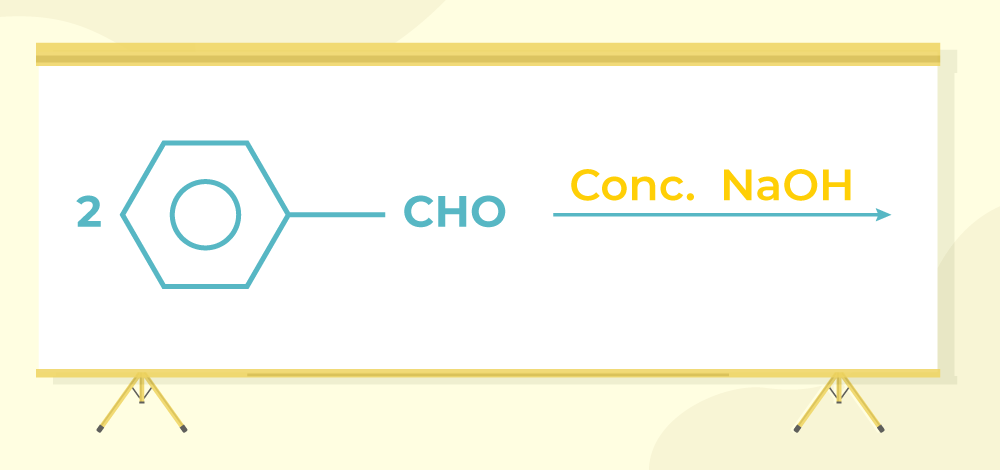### Write the major products of the following reaction,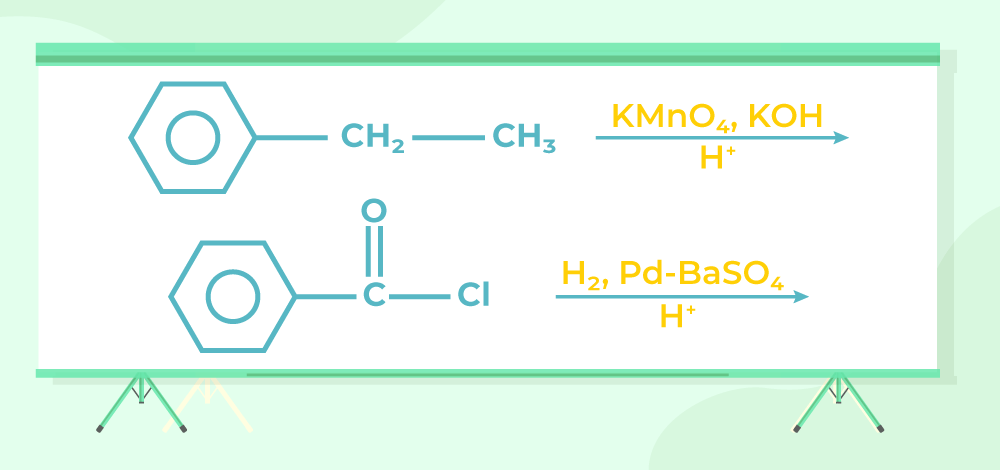(a)

C6H5COCH3 < CH3COCH < CH3CHO < HCHO

• Formaldehyde (HCHO) is the most reactive because it does not have any substituents on the carbonyl carbon, so it can form stable resonance structures easily.
• Acetaldehyde (CH3CHO) has one methyl group attached to the carbonyl group, which makes it less reactive than formaldehyde but more reactive than ketones.
• Acetone (CH3COCH3) has two methyl groups attached to the carbonyl group, which makes it less reactive than both formaldehyde and acetaldehyde.
• Acetophenone (C6H5COCH3) has a bulky phenyl group attached to the carbonyl group, which makes it the least reactive of all the given compounds.

(b)

On heating with Tollens’ reagent, ethanal forms silver mirror whereas propanone does not. Also, ethanal and 1-propanone can be distinguished using the 2,4-DNP (2,4-dinitrophenylhydrazine) test. When 2,4-DNP is added to a solution of ethanal or 1-propanone, a yellow/orange precipitate is formed in both cases. However, the melting point of the precipitate is different for each compound. The melting point of the precipitate formed with ethanal is around 175-178°C, while the melting point of the precipitate formed with 1-propanone is around 95-97°C. By comparing the melting points of the precipitates, we can distinguish between ethanal and 1-propanone.

(c)

Because of resonance by -OH of COOH which reduces the electrophilicity of carboxyl carbon. Carboxylic acids do not undergo nucleophilic addition reactions like aldehydes and ketones because the carboxylic acid group (-COOH) contains both an electron-withdrawing group (the carbonyl group) and an electron-donating group (the -OH group). These two groups have opposing effects on the carbonyl carbon, which makes it less reactive towards nucleophilic addition reactions. In addition, carboxylic acids are more acidic than aldehydes and ketones because the -OH group in the carboxylic acid is more acidic than the -OH group in alcohols. The high acidity of carboxylic acids makes them prone to undergo acid-base reactions rather than nucleophilic addition reactions.

(d) (i)

Due to strong electron withdrawing effect of the carbonyl group: The alpha-hydrogen of aldehydes and ketones are acidic in nature due to the presence of the electron-withdrawing carbonyl group adjacent to the hydrogen atom. This makes the alpha-hydrogen more prone to ionization and thus, more acidic.

Resonance stabilization of the conjugate base: The acidity of the alpha-hydrogen is due to the resonance stabilization of the conjugate base that is formed when the alpha-hydrogen is deprotonated. The negative charge on the conjugate base can be delocalized through resonance into the carbonyl group, stabilizing the negative charge and making it more stable than it would be without the resonance.

(d) (ii)

The products formed here are as follows,

Sodium Benzoate and Benzyl Alcohol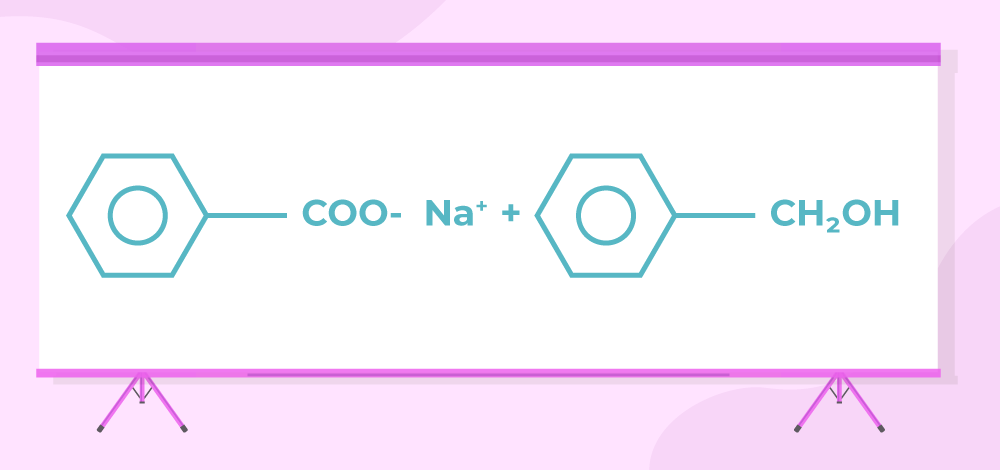Major Products are as follows

Benzoic AcidBenzaldehydeMy Personal Notes arrow_drop_up
Related Tutorials